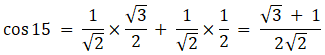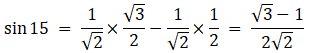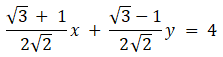• +91 9971497814
• info@interviewmaterial.com

# RD Chapter 23- The Straight Lines Ex-23.7 Interview Questions Answers

### Related Subjects

Question 1 :
Find the equation of a line for which
(i) p = 5, α = 60°
(ii) p = 4, α = 150°

(i) p = 5, α = 60°

Given:

p = 5, α = 60°

The equation of theline in normal form is given by

Using the formula,

x cos α + y sin α= p

Now, substitute thevalues, we get

x cos 60° + y sin 60°= 5

x/2 + √3y/2 = 5

x + √3y = 10

The equation of linein normal form is x + √3y = 10.

(ii) p = 4, α = 150°

Given:

p = 4, α = 150°

The equation of theline in normal form is given by

Using the formula,

x cos α + y sin α= p

Now, substitute thevalues, we get

x cos 150° + y sin150° = 4

cos (180° – θ) =– cos θ , sin (180° – θ) = sin θ

x cos(180° – 30°)+ y sin(180° – 30°) = 4

– x cos 30° + ysin 30° = 4

–√3x/2 + y/2 = 4

-√3x + y = 8

The equation of linein normal form is -√3x + y= 8.

Question 2 : Find the equation of the line on which the length of the perpendicular segment from the origin to the line is 4 and the inclination of the perpendicular segment with the positive direction of x–axis is 30°.

Given:

p = 4, α = 30°

The equation of theline in normal form is given by

Using the formula,

x cos α + y sin α= p

Now, substitute thevalues, we get

x cos 30° + y sin 30°= 4

x√3/2 + y1/2 = 4

√3x + y = 8

The equation of linein normal form is √3x + y = 8.

Question 3 : Find the equation of the line whose perpendicular distance from the origin is 4 units and the angle which the normal makes with the positive direction of x–axis is 15°.

Given:

p = 4, α = 15°

The equation of theline in normal form is given by

We know that, cos15° = cos (45° – 30°) = cos45°cos30° + sin45°sin30°

Cos (A – B) = cos Acos B + sin A sin B

So,And sin 15 = sin(45° – 30°) = sin 45° cos 30° – cos 45° sin 30°

Sin (A – B) = sin Acos B – cos A sin B

So,Now, by using theformula,

x cos α + y sin α= p

Now, substitute thevalues, we get(√3+1)x +(√3-1) y =8√2

The equation of linein normal form is (√3+1)x +(√3-1) y = 8√2.

Question 4 : Find the equation of the straight line at a distance of 3 units from the origin such that the perpendicular from the origin to the line makes an angle α given by tan α = 5/12 with the positive direction of x–axis.

Given:

p = 3, α = tan-1 (5/12)

So, tan α = 5/12

sin α = 5/13

cos α = 12/13

The equation of theline in normal form is given by

By using the formula,

x cos α + y sin α= p

Now, substitute thevalues, we get

12x/13 + 5y/13 = 3

12x + 5y = 39

The equation of linein normal form is 12x + 5y = 39.

Question 5 : Find the equation of the straight line on which the length of the perpendicular from the origin is 2 and the perpendicular makes an angle α with x–axis such that sin α = 1/3.

Given:

p = 2, sin α = 1/3

We know that cos α =√(1 – sin2 α)

= √(1 – 1/9)

= 2√2/3

The equation of theline in normal form is given by

By using the formula,

x cos α + y sin α= p

Now, substitute thevalues, we get

x2√2/3 + y/3 = 2

2√2x + y = 6

The equation of linein normal form is 2√2x + y = 6.

Todays Deals### RD Chapter 23- The Straight Lines Ex-23.7 Contributorskrishan

Name:
Email:

# Latest News# 9000 interview questions in different categories